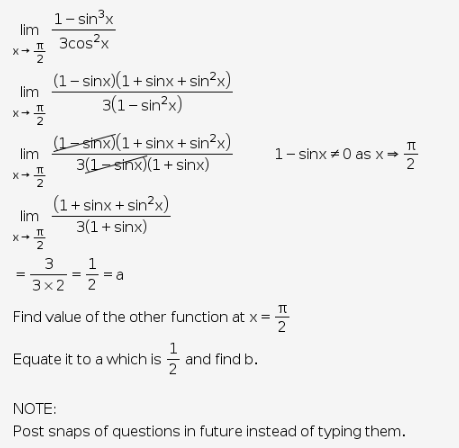# 1-sin^3x/3 cos^2x

• if f(x) = 1-sin3x/3cos2x if x
• a , if x = pi/2 is continous at x = pi/2
• b(1-sinx)/(pi-2x)2

FIND VALUES OF a , b